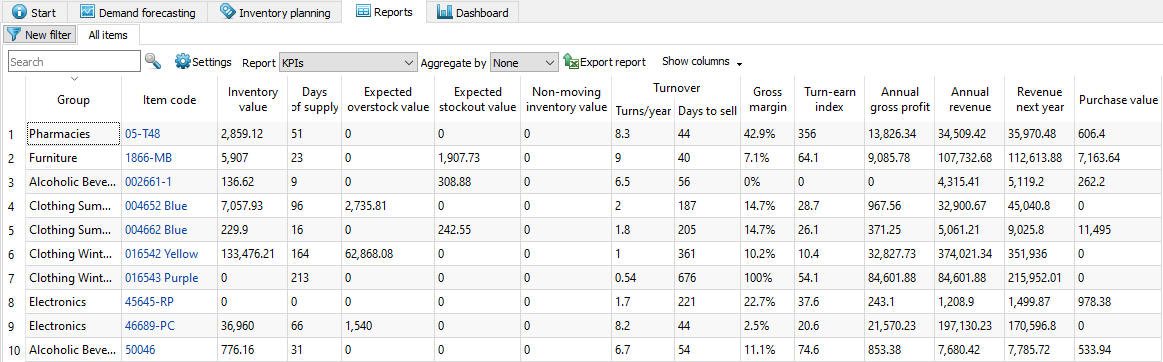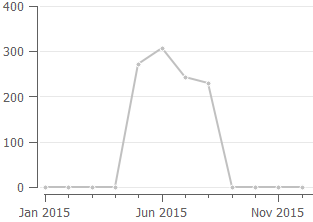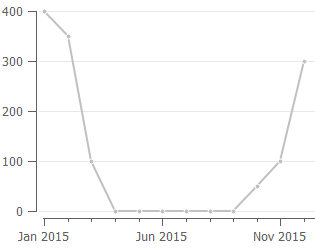kpis-report

# 6.8.3. KPIs Report

KPI (Key Performance Indicators) report shows important indicators of the current project performance. Streamline calculates a variety of indicators. Most of them evaluate the inventory planning process.The table below a brief description of the indicators.

KPI Description
Inventory value Indicates the value of the item in stock
Days of supply Shows how many days of the future demand, starting from the project date, the current On hand (including orders to ship) can cover. You can choose the representation units for this indicator between days or months in the Settings.
Expected overstock value Indicates the value of the expected item overstock
Expected stockout value displays the value of the expected item shortage
Non-moving inventory value Specifies the balance value of the item in stock. It is calculated if the item will not be sold during the forecast horizon period according to the forecast.
Turnover Turns/year Shows how many times an item was purchased and then sold out for the last 12 months
Days to sell Indicates how many days it takes to complete one turnover cycle
Gross margin Displays a gross profit margin for the item
Turn-earn index Specifies an item gross margin accumulated over the last 12 months
Annual gross profit Shows an aggregation of the transactions' profit over the last 12 complete months
Annual revenue Shows the averaged item revenue over the last 12 months
Revenue next year Indicates the expected item revenue for the next 12 months according to the forecasts
Purchase value Displays the purchase amount for each planning item

Streamline allows for changing the way the Inventory value and Non-moving inventory value KPIs are calculated. You can set Streamline to account for the To receive quantity of purchase orders when it calculates these in the Settings.

2019/10/16 10:38 ·

Below we give a more detailed description of these KPIs.

## Item Value-Dependent Indicators

There is a set of KPIs that depends on the item value. Item value is a characteristic that is taken as Inventory value/unit or Purchase price/unit data type. If both data types are given, the former is taken as the item value.

Item value-dependent indicators are the following:

Below we explain how they are calculated.

### Inventory Value

The Inventory value indicator shows the value of the item in stock. If it is not imported directly using the Aggregated spreadsheet connection, it is calculated as:

Inventory value = item value * On hand.

### Expected Overstock Value

The Expected overstock value indicator indicates the expected overstock value for an item. It is calculated as:

Expected overstock value = item value * overstock_qty.

### Non-Moving Inventory Value

The Non-moving inventory value indicator is calculated for those items that will not be sold during the forecast horizon according to the forecast. Streamline doesn't computes this KPI for items that are forecasted using an intermittent demand model. The formula for this indicator is:

Non-moving inventory value = item value * On hand.

### Gross Margin

The Gross margin indicator is the monthly gross profit margin. Its calculation is based on the compound interest and the formula looks like:,

inflationCoefficient = 1 + interest rate,

selloutTime = (lead time + Days to sell) / 365.25,

where:

• avgSalesPrice – the average selling price for the current month;
• lead time – is the Lead time or DC lead time depenting on the echelon the item resides at;
• interest rate – the annual interest rate that is taken from the Settings

### Annual Gross Profit

This indicator is an aggregation of transactions' profit over the last 12 complete months. It is calculated in two ways depending on the available data:

1. It is the sum of the Transaction profit imported from the data source.
2. It is calculated using the formula:,

where:

• N – the number of data aggregation periods making up 12 months;
• avg sales pricet – the average sales price over the `t`-th period. It can be calculated if the Sales price/unit data type was imported;
• salest – the item quantity sold in the `t`-th period.

Streamline uses the first method primarily.

## Expected Stockout Value

The Expected stockout value indicator is the expected inventory deficiency value in the base currency. It is calculated as:

Expected stockout value = avg sales price * stockout_qty,

where avg sales price is the average sales price in the current period. If we don't have sales in the current period, data of the previous period is used.

## Turnover

Inventory turnover tells how many times an item was purchased and then sold out over a particular period of time. Its formula looks like:,

where:

• N – the number of days in the period;
• Salesi – the item quantity sold on `i`-th day;
• K – the number of days in the period without stockout;
• On handi – stock on hand at the end of `i`-th day. It is determined based on On hand change or On hand (as remaining after a transaction) imported from the data source.

If sales history can't cover the period, it is brought to it proportionally using the formula:where:

• N – the number of days in the period;
• K – the sales history length in days;
• Salesi – the item quantity sold on `i`-th day.

The Turns/year column of this report shows the turnover over the last 12 months.

## Turn-earn Index

This indicator shows an item gross margin accumulated over the last 12 months and calculated as:

Turn-earn index = Gross margin * Turns/year.

This indicator is usually used to rank products and find the most profitable items.

## Annual Revenue

Let’s explain how this indicator is calculated. Consider the product sales history depicted in the figure below.To calculate annual revenue in this case, Streamline always skips zero demand before the sales have started. So only the sales of the last 8 months are taken into account. The formula looks like:

Annual revenue = RevenueMay-Dec / 8 * 12

If we have sales history shown in the figure below, then sales of all the months are taken into account:

Annual revenue = RevenueJan-Dec / 12 * 12So, as you see, this indicator depends on the sales history of the last 12 months, and thus, may significantly change from month to month.

Revenue data of the current period is taken into account only if this period is complete. Thus, Annual revenue accounts for the data of the last 12 complete months.

## Revenue Next Year

If the forecast horizon is one year, the next year's revenue is the summed revenue of the next 12 months. Otherwise, it is calculated proportionally using the available data. For example, if data aggregation period is one month, this indicator is calculated as:

Revenue next year = Revenuen /n * 12,

where:

• n is the number of months to forecast ahead;
• Revenuen is summed revenue over the future n months.

## Purchase Value

This indicator shows the purchase amount for each planning item. It is calculated based on the current purchase recommendations. This column allows you to view the total purchase amount by each supplier if you group this report by the Supplier using the Aggregate by drop-down.

kpis-report.txt · Last modified: 2021/01/07 14:23 by admin

### Page Tools